;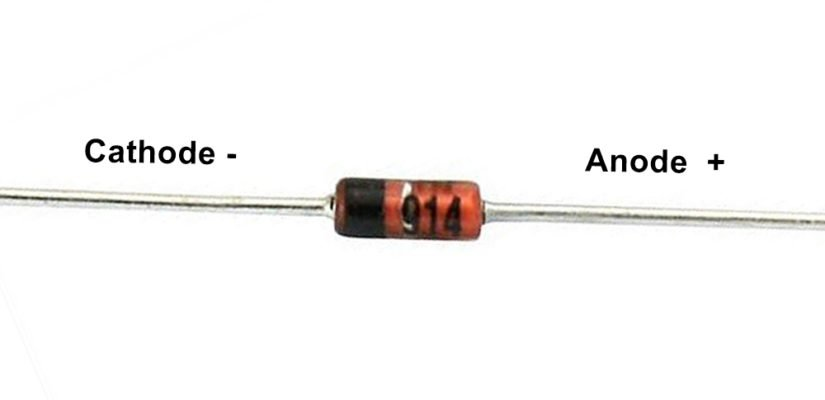## Zener diode

Zener diode is a type of diode which flow of current also in reverse bias and in applications it always connected in reverse bias and zener diode limit the voltage, this diode is used for regulating the voltage in any circuit. It always works in zener breakdown voltage. Zener breakdown voltage is voltage level at this zener diode starts the current conduction in reverse bias. When reverse voltage increases then current conduction starts at a level, this level of voltage called breakdown voltage and the current flowing through diode is called zener current. This process is called avalanche breakdown .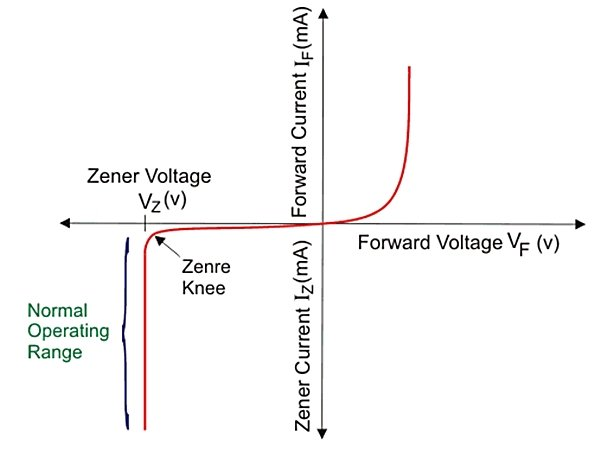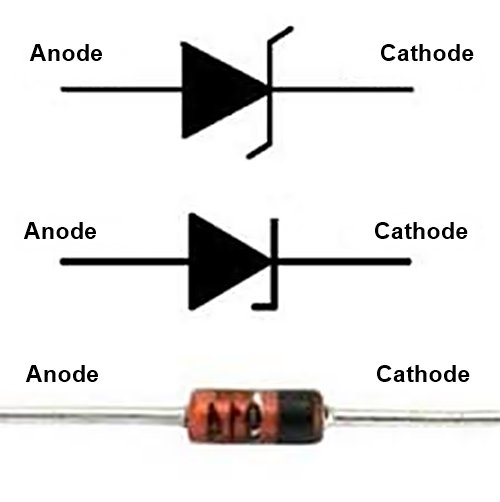First we take a look on normal diode . A normal diode works only in forward bias, means voltage on cathode is less than anode .  Voltage on anode is high with respect of cathode then it is called forward bias, in this condition a norml diode starts conduction. If voltage on anode is 2v and voltage on cathode is 0v or voltage on anode is 5v and 2v on cathode then conduction starts and diode will work , but if voltage on anode is 0v and voltage is on cathode is 2v then normal diode will not work and this is called reverse connection.

In reverse bias after a limitation of current flow barrier break and a reverse current flow

A normal diode only work in forward bias . If we give voltage more than breakdown voltage in reverse bias in normal diode then it will damage permanently. This drawback of normal diode removed in zener diode . A zener diode work as normal diode If it connected in forward bias . But zener diode also conducts current in reverse bias connection with specified condition.

### zener diode as voltage regulator

We try to understand how zener diode works and regulate the voltage.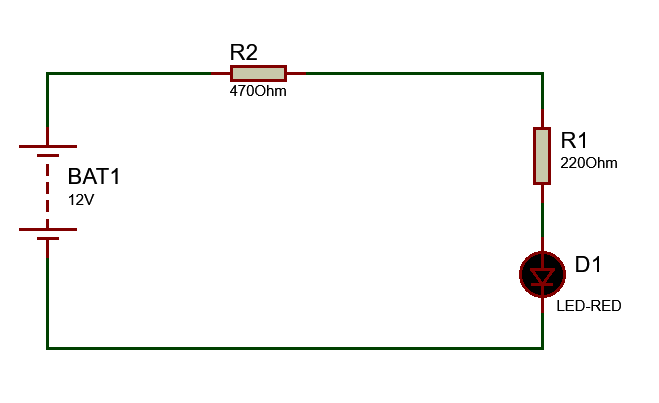V1 (BAT1) is a DC source , a load is connected and denoted as RL, a zener diode is connected across the RL through a resistor . In this circuit anode of zener diode is connected with negative terminal of battery and cathode of zener is connected with positive point of battery. So this connection is in reverse bias. 12v DC source or battery applied and R1 is 220 ohm , R2 is 470 ohm and an LED is connected with R1 as Load.

Now we check the voltage at all resistors and load, voltage are divided at all resistor and load according to resistances at each point as divider formula. I connect three DC voltmeter at each resistor and load to measure the Voltage Drop at each part. As you see in the circuit diagram given Voltage drop across R1 = 3.114v, across R2= 6.65v and voltage drop at load (LED)=2.24v. so, 3.11v+6.65v+2.24v+6.65v=12v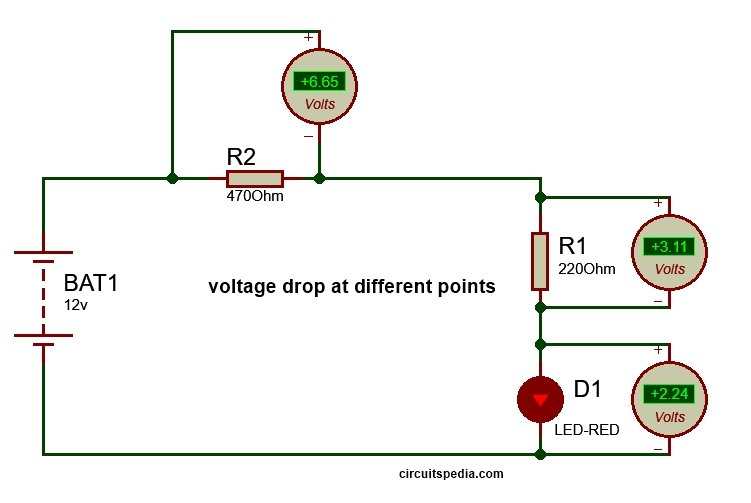In this circuit arrangement the Total voltage across the load is 2.24v (Here load is LED). If i want to voltage at load load fix 3v But input source voltage remain 12v, then what should i do ?

### zener diode circuitFor 3v output we should connect a 3v zener diode to parallel with output load . One thing is noted here that a resistor must connect before the zener as series with positive voltage . But here is a Question about this resistor , Why use this resistor ?

### Why do i connect a resistor with zener diode ?

When we connect zener diode at output in reverse bias , then current through diode pass according to the source voltage. Here source voltage is 12v that is fix . If the source voltage increase then the current conduction through zener diode also increases and extra voltage drop  at the resistor R2. If the source voltage vary then the current conduction through zener diode and resistor also vary and all varied extra voltage will drop at resistor R2 and we found a fix regulated voltage at output. So all extra voltage will drop at resistor that is connected in series with zener. It means according to applied input voltage increases, the zener diode allow to pass more current through itself by the series resistor therefore the current conduction through resistor and zener diode increase as per input voltage, and the point of zener diode we get regulated fix voltage as its rating.

If you need 3v output then use 3v or 3.1v zener diode and according to if you need 5v then use 5.1v  and 9v fzener for 9v output 12v zener for 12v output.....But applied input voltage Must higher than required output voltage because zener diode always works in breakdown region so for breakdown the applied voltage should higher than the breakdown voltage.

There are limitation of current conduction capacity of each zener . Zener diode available in market at the rating of  different watt with different voltages.

#### The value of series resistor can be determined by using following given formula

R=(Vin -Vz)/Iz

Where R= Series resistor

Vin= Applied input voltage

Vz= Zener voltage (this is equal to Output voltage V0)

Iz= Zener current